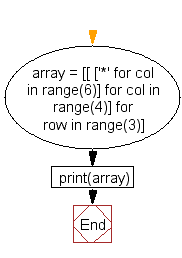﻿ Python: Generate a 3D array - w3resource# Python: Generate a 3D array

## Python List: Exercise - 13 with Solution

Write a Python program to generate a 3*4*6 3D array whose each element is *.

Sample Solution:-

Python Code:

``````array = [[ ['*' for col in range(6)] for col in range(4)] for row in range(3)]
print(array)
```
```

Sample Output:

```[[['*', '*', '*', '*', '*', '*'], ['*', '*', '*', '*', '*', '*'], ['*', '*', '*', '*', '*', '*'], ['*', '*', '
*', '*', '*', '*']], [['*', '*', '*', '*', '*', '*'], ['*', '*', '*', '*', '*', '*'], ['*', '*', '*', '*', '*'
, '*'], ['*', '*', '*', '*', '*', '*']], [['*', '*', '*', '*', '*', '*'], ['*', '*', '*', '*', '*', '*'], ['*'
, '*', '*', '*', '*', '*'], ['*', '*', '*', '*', '*', '*']]]
```

Pictorial Presentation:Flowchart:## Visualize Python code execution:

The following tool visualize what the computer is doing step-by-step as it executes the said program:

Python Code Editor:

Have another way to solve this solution? Contribute your code (and comments) through Disqus.

What is the difficulty level of this exercise?

Test your Python skills with w3resource's quiz

﻿

## Python: Tips of the Day

```print(2_000_000)
```2000000